# Two planes

Two planes flying from airports A and B, 420 km distant against each other. Plane from A took off 15 minutes later and flies at an average speed of 40 km/h higher than the plane from B. Determine the average speed of this two aircraft if you know that it will meet 30 minutes after takeoff A.

Result

a =  360 km/h
b =  320 km/h

#### Solution:

a=b+40
a*30/60 + b* (45/60)=420

a-b = 40
30a+45b = 25200

a = 360
b = 320

Calculated by our linear equations calculator.

Leave us a comment of example and its solution (i.e. if it is still somewhat unclear...):

Showing 0 comments:Be the first to comment!#### To solve this verbal math problem are needed these knowledge from mathematics:

Do you have a linear equation or system of equations and looking for its solution? Or do you have quadratic equation?

## Next similar examples:

1. Journey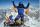The road from A to B measures 11.5 km. Firstly up the hill, then by level plane and then downhill. Tourist goes uphill at 3 km/h, on the plane 4 km/h and downhill 5 km/h. From point A to B went 2h 54 min back 3h 6 min. How long is the segment of level pla
2. Walkers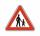From points A and B simultaneously started against each other two walkers. After meeting both continue to B. Second walker came to B 2 hours before the first walker. It's speed is 2.7 times of speed of the first pedestrian. How many hours went pedestria
3. Distance between two citiesCar went from A to B 4h. On the way back, the car was up 15km / h faster. The return trip took 48 minutes. Shorter than the way there. Find the distance of the cities A and B.
4. Theorem proveWe want to prove the sentense: If the natural number n is divisible by six, then n is divisible by three. From what assumption we started?
5. Ball gameRichard, Denis and Denise together scored 932 goals. Denis scored 4 goals over Denise but Denis scored 24 goals less than Richard. Determine the number of goals for each player.
6. Warehouses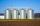In the three warehouses, a total of 70 tons of grain was stored. In the second warehouse was stored 8.5t less and in the third 3.5t more than in the first. How many tons of grain was stored in each warehouse?
7. Linsys2Solve two equations with two unknowns: 400x+120y=147.2 350x+200y=144
8. PoojaPooja and Deepa age is 4:5, 4 years back it was 8:11. What is the age of Pooja now?
9. ChildrenThe group has 42 children. There are 4 more boys than girls. How many boys and girls are in the group?
10. Geometric sequence 5About members of geometric sequence we know: ? ? Calculate a1 (first member) and q (common ratio or q-coefficient)
11. Journey to school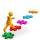On the way to school I went steady step. In the first half of the journey, I counted every second step, in the second half every third. How many steps I do when I went to school if I counted double step are 250 more than three steps?
12. Father 7Father is 6 times older than his son. After 4 years, the father will only be 4 times older. What are their present ages?
13. WavelengthCalculate the wavelength of the tone frequency 11 kHz if the sound travels at speeds of 343 m/s.
14. Father and sonWhen I was 11, my father was 35 years old. Today, my father has three times more years than me. How old is she?
15. FamilyFamily has 4 children. Ondra is 3 years older than Matthew and Karlos 5 years older than the youngest Jane. We know that they are together 30 years and 3 years ago they were together 19 years. Determine how old the children are.
16. Null points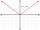Calculate the roots of the equation: ?
17. Lee isLee is 8 years more than twice Park's age, 4 years ago, Lee was three times as old. How old was Lee 4 years ago?